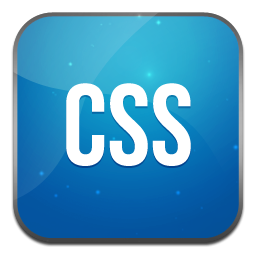# 条件注释判断浏览器版本

• 2
• 2,654 ℃
• A+<!--[if !IE]><!--> 除IE外都可识别 <!--<![endif]-->

<!--[if IE]> 所有的IE可识别 <![endif]-->

<!--[if IE 6]> 仅IE6可识别 <![endif]-->

<!--[if lt IE 6]> IE6以及IE6以下版本可识别 <![endif]-->

<!--[if gte IE 6]> IE6以及IE6以上版本可识别 <![endif]-->

<!--[if IE 7]> 仅IE7可识别 <![endif]-->

<!--[if lt IE 7]> IE7以及IE7以下版本可识别 <![endif]-->

<!--[if gte IE 7]> IE7以及IE7以上版本可识别 <![endif]-->

<!--[if IE 8]> 仅IE8可识别 <![endif]-->

<!--[if IE 9]> 仅IE9可识别 <![endif]-->

! [if !IE] The NOT operator. This is placed immediately in front of the featureoperator, or subexpression to reverse the Boolean meaning of the expression.
NOT运算符。这是摆立即在前面的功能操作员，或子表达式扭转布尔表达式的意义。
lt [if lt IE 5.5] The less-than operator. Returns true if the first argument is less than the second argument.

lte [if lte IE 6] The less-than or equal operator. Returns true if the first argument is less than or equal to the second argument.

gt [if gt IE 5] The greater-than operator. Returns true if the first argument is greater than the second argument.

gte [if gte IE 7] The greater-than or equal operator. Returns true if the first argument is greater than or equal to the second argument.

( ) [if !(IE 7)] Subexpression operators. Used in conjunction with boolean operators to create more complex expressions.

& [if (gt IE 5)&(lt IE 7)] The AND operator. Returns true if all subexpressions evaluate to true
AND运算符。如果所有的子表达式计算结果为true，返回true
| [if (IE 6)|(IE 7)] The OR operator. Returns true if any of the subexpressions evaluates to true.
OR运算符。返回true，如果子表达式计算结果为true。

<!--[if lt IE 9]>

<!--[else]>

<![endif]-->

<!--[if lt IE 9]>

<![endif]-->

• 我的微信
• 加我请备注缘由
•• 我的微信公众号
• 轻松随便关注
•## 目前评论：2   其中：访客  1   博主  1

•Sawyer 1

许多古老的浏览器可以不管他了

•陈 学虎 Admin

是的。